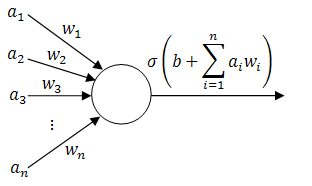# Backpropagation Tutorial

The PhD thesis of Paul J. Werbos at Harvard in 1974 described backpropagation as a method of teaching feed-forward artificial neural networks (ANNs). In the words of Wikipedia, it lead to a "rennaisance" in the ANN research in 1980s.

As we will see later, it is an extremely straightforward technique, yet most of the tutorials online seem to skip a fair amount of details. Here's a simple (yet still thorough and mathematical) tutorial of how backpropagation works from the ground-up; together with a couple of example applets. Feel free to play with them (and watch the videos) to get a better understanding of the methods described below!

Training a single perceptron

Training a multilayer neural network

##### 1. Background

To start with, imagine that you have gathered some empirical data relevant to the situation that you are trying to predict - be it fluctuations in the stock market, chances that a tumour is benign, likelihood that the picture that you are seeing is a face or (like in the applets above) the coordinates of red and blue points.

We will call this data training examples and we will describe $i$th training example as a tuple $(\vec{x_i}, y_i)$, where $\vec{x_i} \in \mathbb{R}^n$ is a vector of inputs and $y_i \in \mathbb{R}$ is the observed output.

Ideally, our neural network should output $y_i$ when given $\vec{x_i}$ as an input. In case that does not always happen, let's define the error measure as a simple squared distance between the actual observed output and the prediction of the neural network: $E := \sum_i (h(\vec{x_i}) - y_i)^2$, where $h(\vec{x_i})$ is the output of the network.

#### 2. Perceptrons (building-blocks)

The simplest classifiers out of which we will build our neural network are perceptrons (fancy name thanks to Frank Rosenblatt). In reality, a perceptron is a plain-vanilla linear classifier which takes a number of inputs $a_1, ..., a_n$, scales them using some weights $w_1, ..., w_n$, adds them all up (together with some bias $b$) and feeds everything through an activation function $\sigma \in \mathbb{R} \rightarrow \mathbb{R}$.

A picture is worth a thousand equations:Perceptron (linear classifier)

To slightly simplify the equations, define $w_0 := b$ and $a_0 := 1$. Then the behaviour of the perceptron can be described as $\sigma(\vec{a} \cdot \vec{w})$, where $\vec{a} := (a_0, a_1, ..., a_n)$ and $\vec{w} := (w_0, w_1, ..., w_n)$.

To complete our definition, here are a few examples of typical activation functions:

• sigmoid: $\sigma(x) = \frac{1}{1 + \exp(-x)}$,
• hyperbolic tangent: $\sigma(x) = \tanh(x)$,
• plain linear $\sigma(x) = x$ and so on.

Now we can finally start building neural networks. Continue reading

# Conway's Game of Life

#### 1. Description

In 1970s John Horton Conway (British mathematician and University of Cambridge graduate) opened a whole new field of mathematical research by publishing a revolutionary paper on the cellular automaton called the Game of Life. Suffice it to say that the game which he has described with four simple rules has the power of a universal Turing machine, i.e. anything that can be computed algorithmically can be computed within Conway's Game of Life (outlines of a proof for given by Berlekamp et al; implemented by Chapman as a universal register machine within the Game of Life in 2002).

The Game of Life is a zero-player game, i.e. the player interacts only by creating an initial configuration on a two-dimensional grid of square cells and then observing how it evolves. Every new generation of cells (which can be either live or dead) is a pure function of the previous generation and is described by this set of rules:

1. Any live cell with fewer than two live neighbours dies, as if caused by underpopulation.
2. Any live cell with more than three live neighbours dies, as if by overcrowding.
3. Any live cell with two or three live neighbours lives on to the next generation.
4. Any dead cell with exactly three live neighbours becomes a live cell.

For more information, patterns and current news about the research involving Game of Life check out the brilliant LifeWiki at conwaylife.com.

#### 2. Implementation

The following applet visualising the Game of Life has been developed as part of the coursework for Object-Oriented Programming at the University of Cambridge, all code was written and compiled in Sun's Java SE 1.6.

Click on any of the screenshots or the button below to launch the Game of Life (and if nothing shows up, make sure that you have the Java Runtime Environment (JRE) installed).